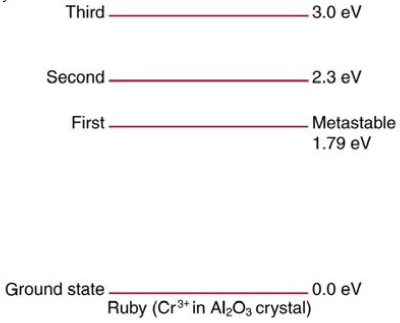Chapter 30, Problem 32PE

Chapter
Section
Textbook Problem

Ruby lasers have chromium atoms doped in an aluminum oxide crystal. The energy level diagram for chromium in a ruby is shown in Figure 30.64. What wavelength is emitted by a ruby laser?Figure 30.64 Chromium atoms in an aluminum oxide crystal have these energy levels, one of which is metastable. This is the basis of a ruby laser. Visible light can pump the atom into an excited state above the metastable state to achieve a population inversion.

To determine

The wavelength emitted by ruby laser when Ruby lasers have chromium atoms doped in an aluminum oxide crystal.

Explanation

Given Data:

Given energy-level diagram for chromium in a ruby.

Formula Used:

Energy is given as

E=hcλ

Where, E= Energy

h= Planck's constant

c= Speed of light

λ= Wavelength

Energy released during transition between metastable state and ground state is given as

ΔE=EmEg

Where ΔE= Energy released during the transition between two states

Em= Energy of meta- stable state

Eg= Energy of ground state

Calculation:

From energy level diagram,

Em=1.79eV

Eg=0eV

Energy released during transition is calculated as

ΔE=(1.79eV)(0eV)

ΔE=1.79eV

Now, wavelength emitted by ruby laser is calculated using Planck's equation

We have h=6.626×1034J.s

E=1.79eV

c=3×108m/sec

Substituting the values in equation of energy, we have

E=hcλ

1

Still sussing out bartleby?

Check out a sample textbook solution.

See a sample solution

The Solution to Your Study Problems

Bartleby provides explanations to thousands of textbook problems written by our experts, many with advanced degrees!

Get Started

Find more solutions based on key concepts